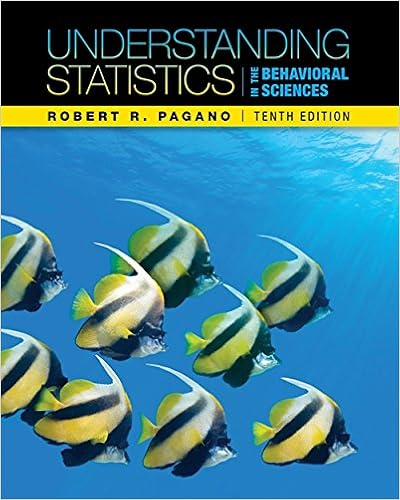# 2 repeat step 1 for each of the 3 vertexes of the

• Notes
• 175

This preview shows page 40 - 44 out of 175 pages.

##### We have textbook solutions for you!
The document you are viewing contains questions related to this textbook.The document you are viewing contains questions related to this textbook.
Chapter 8 / Exercise 8
Understanding Statistics in the Behavioral Sciences
PaganoExpert Verified
(2) Repeat Step 1 for each of the 3 vertexes of the triangle. (3) The creases made in Steps 1 and 2 above should meet at the incenter of the triangle. 34
##### We have textbook solutions for you!
The document you are viewing contains questions related to this textbook.The document you are viewing contains questions related to this textbook.
Chapter 8 / Exercise 8
Understanding Statistics in the Behavioral Sciences
PaganoExpert Verified
CHAPTER 2. TOPICS IN PLANE GEOMETRY What is it really? If we draw a circle inside the triangle, such that the circle touches each of the sides of the triangle, then the incenter is the center of this circle. This circle is sometimes called the incircle . The Centroid Theorem 12 The lines of a triangle which connect the vertexes to the mid- points of the opposite sides of a triangle meet at a point. This point is called the centroid . The line segments used in finding the centroid have a special name: Definition A median of a triangle is a line segment which connects a vertex to the midpoint of the opposite side. Paper-Folding Construction To construct the centroid using paper-folding, perform the following steps: (1) Fold the leg of the triangle over top of itself so that its endpoints meet. (2) Take the vertex opposite the leg above, and make a crease that starts at the vertex and extends to where the crease made in Step 1 meets the leg opposite the vertex. (3) Repeat Steps 1 and 2 for each of the 3 legs of the triangle. (4) The creases made in the paper in Steps 2 and 3 above should meet at the centroid of the triangle. 35
2.1. TRIANGLES What is it really?
Question Is it always/ever the case that the circumcenter, the orthocenter, the incenter, and the centroid are all actually the same point? ? Question Can the circumcenter ever be outside the triangle? ? Question Can the orthocenter ever be outside the triangle? ? Question Can the incenter ever be outside the triangle? ? Question Can the centroid ever be outside the triangle? ? Keeping all these ideas straight can be tough, and unfortunately, I don’t know of an easy way to learn them. However, a first step is knowing the names of each of the centers described above. Here is simple mnemonic that may help: c e C O I N i r n t r t c r c h e o u o n i m c t d c e e e n r n t t e e r r 36
CHAPTER 2. TOPICS IN PLANE GEOMETRY Note that the centers that correspond to the IN part of COIN are in side the triangle! 2.1.2 Theorems about Triangles Now consider some triangle and look at the orthocenter, the centroid, and the circumcenter. In the illustration below, O is the orthocenter, N is the centroid, and C is the circumcenter: N C O Do you notice anything? Theorem 13 (Euler) The circumcenter, the centroid, and the orthocenter are on a line. The centroid lies a third of the distance from the circumcenter to the orthocenter. This line is called the Euler line .
•••